# One-Diode Model

Given the 1-diode model parameters for the module, this routine computes the module’s current-voltage characteristics. Figure 35 shows the equivalent circuit of a PV cell. The following algorithm includes the recombination current, Irec, a refinement in the model that can be set to zero if the parameters required to determine the current (μ, τeff, di, Vbi) are not provided in the module definition input file.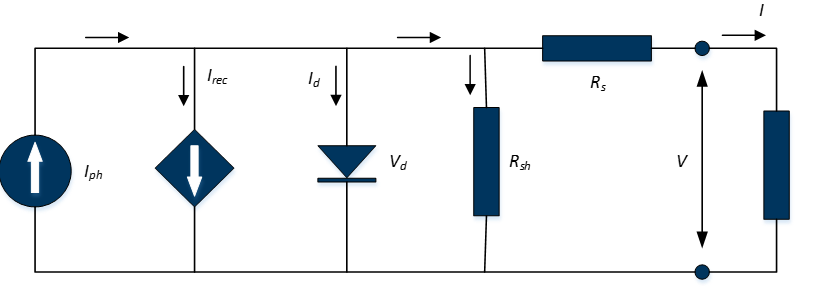## Inputs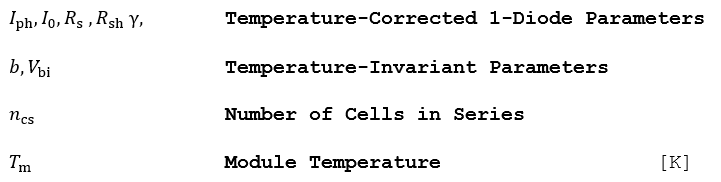## Outputs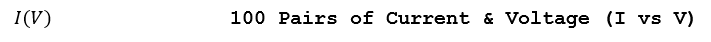## Algorithm

1.) Solve the following transcendental equation that relates the module’s output current i and output voltage υ to the photocurrent Iph, and the 1-diode parameters corrected for the temperature and irradiance level (I0, Rsh, Rs, γ).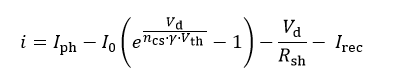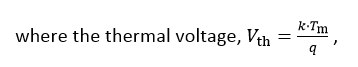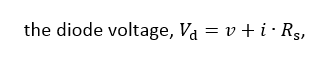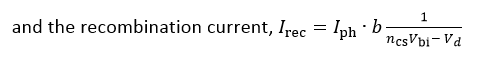The parameter b, given in the module parameter definition, combines the following terms: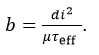Expanded, the transcendental function for the output current becomes: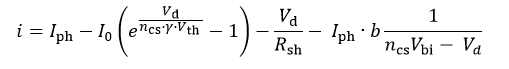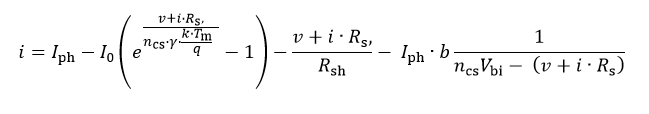2.) The current should be solved for diode voltages in the interval [0, νoc,g], where νoc,g is the guess for the open-circuit voltage, and can be found by setting the I to zero in the above equation (neglecting Irec):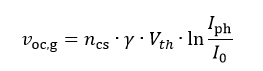3.) The limits of the I(V) curve in the first quadrant are defined by the open-circuit voltage and short-circuit current, which can be found by setting i and ν to 0, respectively.

4.) Find the maximum power point diode voltage using the Newton-Raphson method by iteratively solving the following equation until the difference between Vd,n+1 and νd,n is arbitrarily small.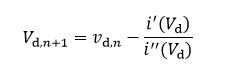5.) Find the maximum power point current by solving the 1-diode equation.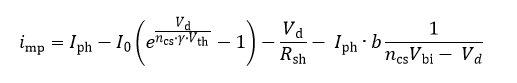6.) Find the maximum power point voltage at the output terminal of the equivalent circuit by subtracting the voltage drop across the series resistance.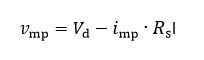## Reference

Mermoud, A., Conception et dimensionnement de systèmes photovoltaïques : Introduction des modules PV en couches minces dans le Logiciel PVsyst. Université de Genève, 2005.